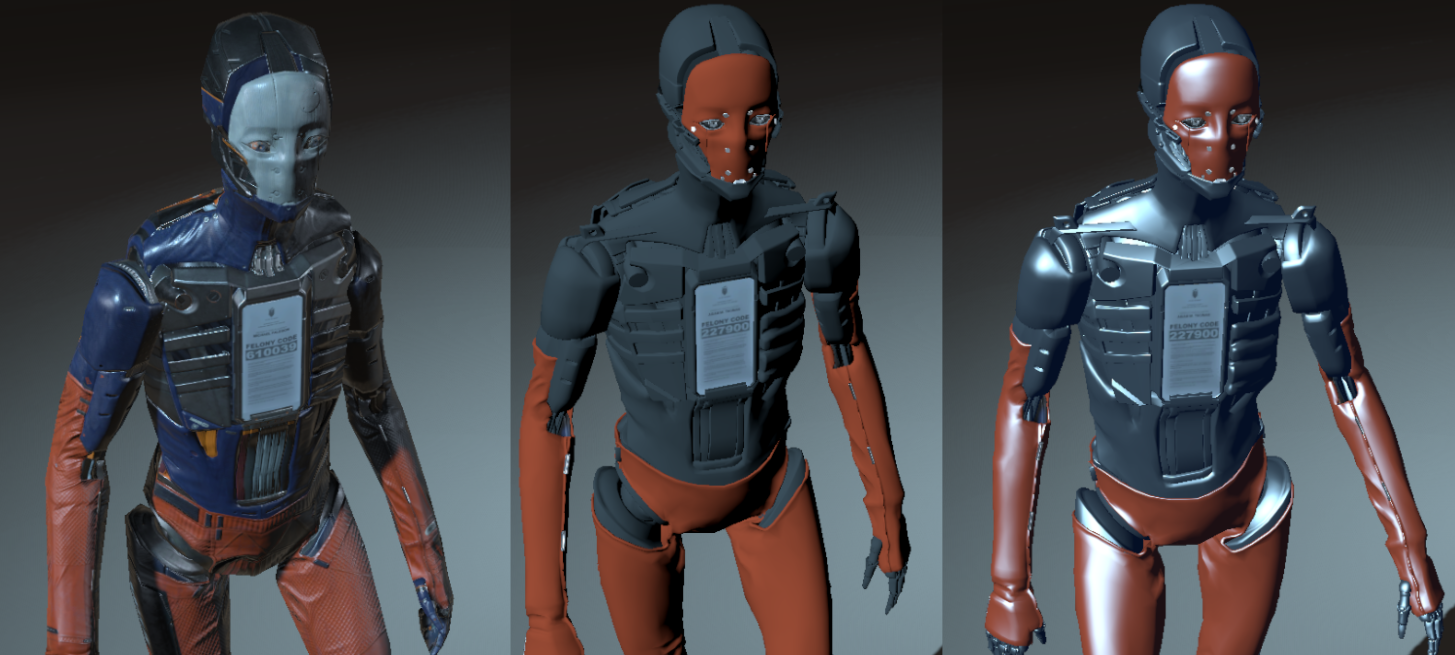# 经验光照模型## 约定

• $$L$$：(被观察点到光源的)光线向量 (入射光的反方向)
• $$R$$：反射向量
• $$N$$：法线向量
• $$V$$：(被观察点到观察点的)视线向量
• $$T$$：切线向量
• $$H$$：半角向量

\begin{aligned} R&=2(N\cdot L)N-L \\ H&={V+L\over\|V+L\|} \end{aligned}

## Lambert

$C_{Lambert}=Albedo\times LightColor\times(N\cdot L)$

$C_{Lambert}'=Albedo\times LightColor\times\max(0,N\cdot L)$

## Half-Lambert

$C_{HalfLambert}=Albedo\times LightColor\times(0.5\times N\cdot L+0.5)^2$

## Phong

Phong模型假设物体的最终着色表现由四部分组成

$C_{Phong}=C_{emission}+C_{ambient}+C_{specular}+C_{diffuse}$

$C_{diffuse}=Albedo_{diffuse}\times LightColor\times(N\cdot L)$

emission为物体的自发光部分，这部分贡献直接来自物体的自发光颜色，在这里，自发光物体并不会被当做光源参与其他计算：

$G_{emission}=M_{emission}$

ambient为环境光部分，这也是历史上首次涉及全局光的一个模型 (虽然只是简单的一项加法)，这个模型假设当前场景存在一个环境颜色，并在所有着色计算中都会加上这一项：

$C_{ambient}=G_{ambient}$

specular为高光部分，这是基于对金属类材质的高光表现而添加一部分贡献，根据经验，我们的视线越接近反射方向，我们看到的高光反射就越明显，因此也就有：

$C_{specular}=Albedo_{specular}\times LightColor\times \max(0,V\cdot R)^{Gloss}$

## Blinn-Phong

Blinn-Phong模型是针对Phong模型中高光计算部分提出的另一种实现。它基于另一个观察：当视线越接近反射方向时，半角向量也越接近法线，因此也就有：

$C_{specular}=Albedo_{specular}\times LightColor\times \max(0,N\cdot H)^{Gloss}$